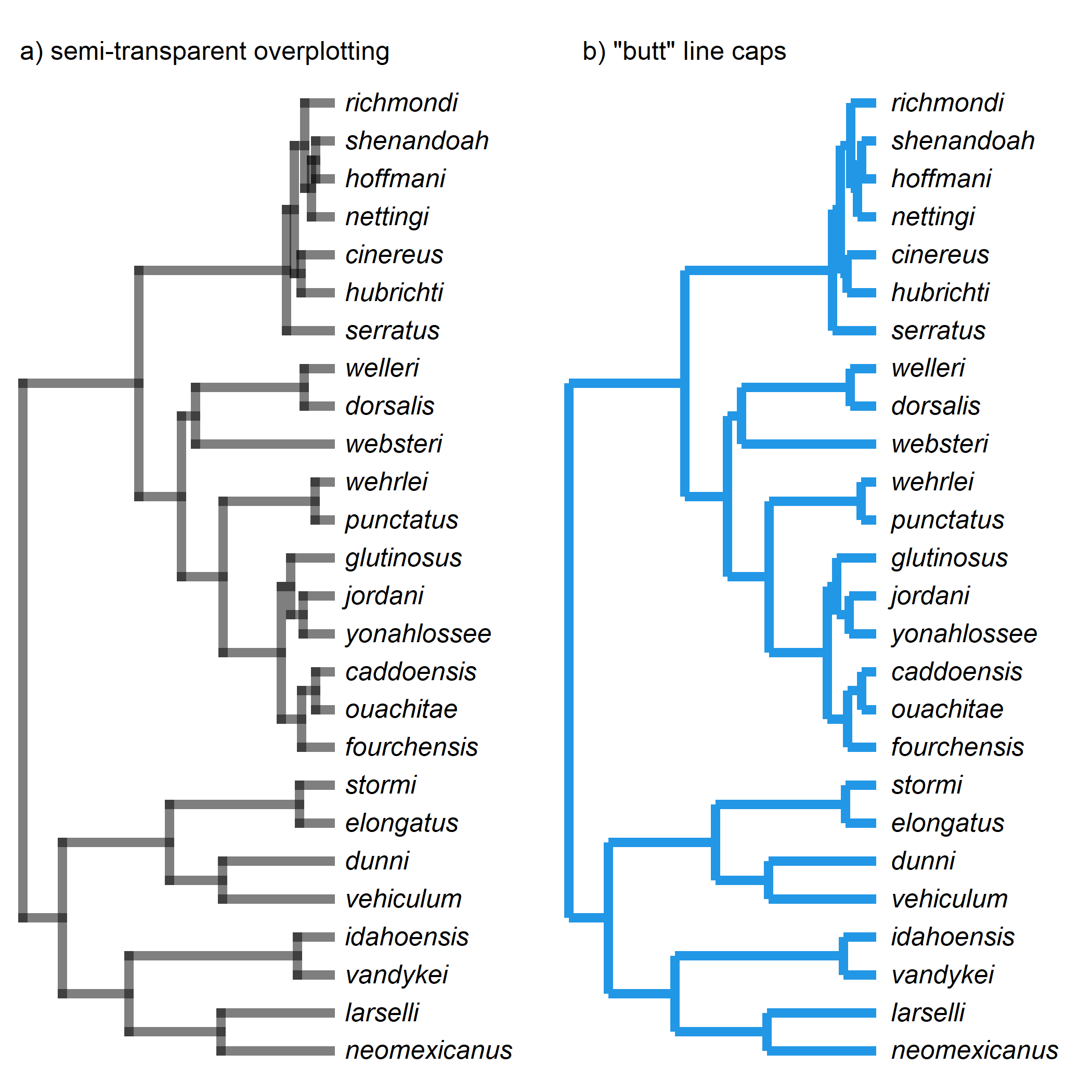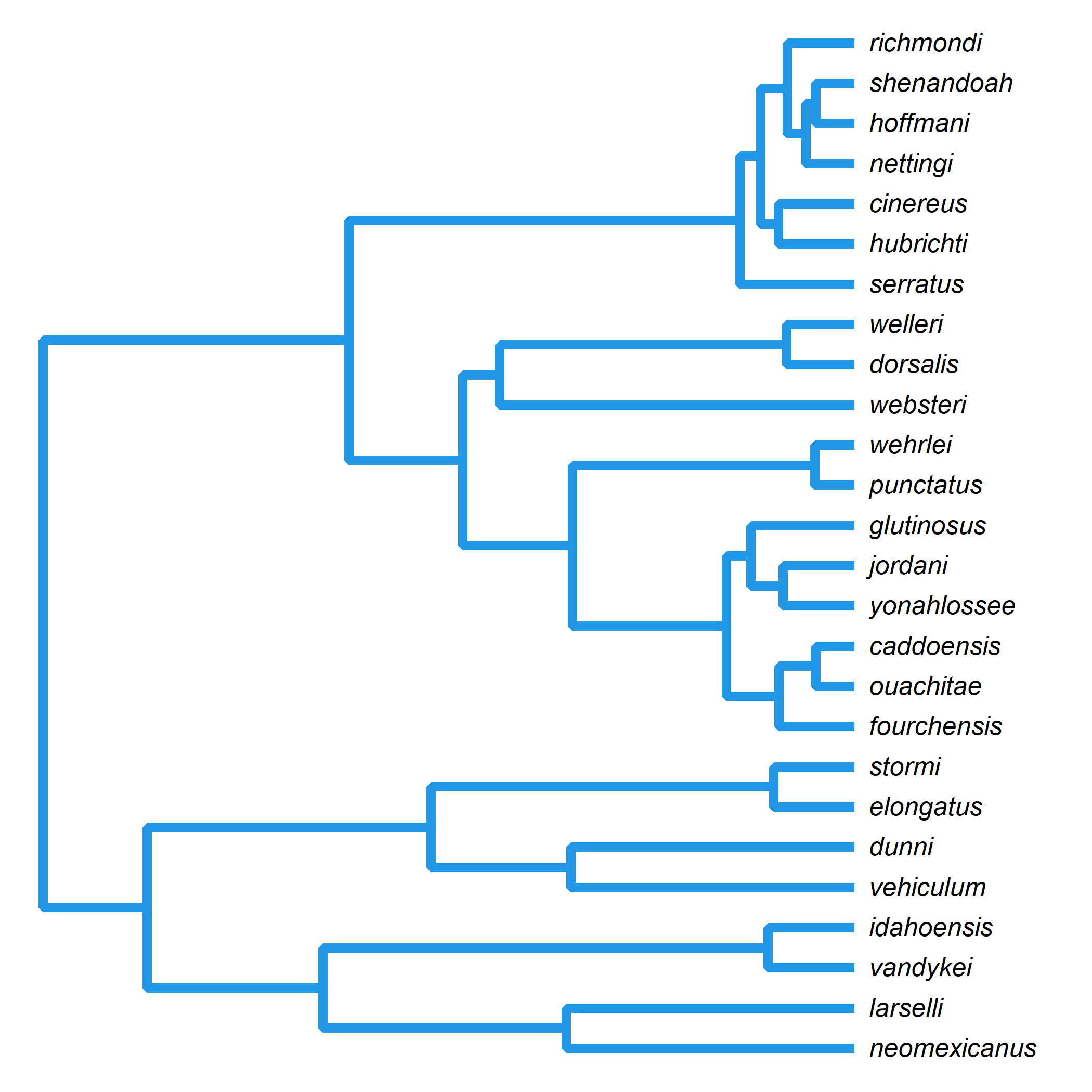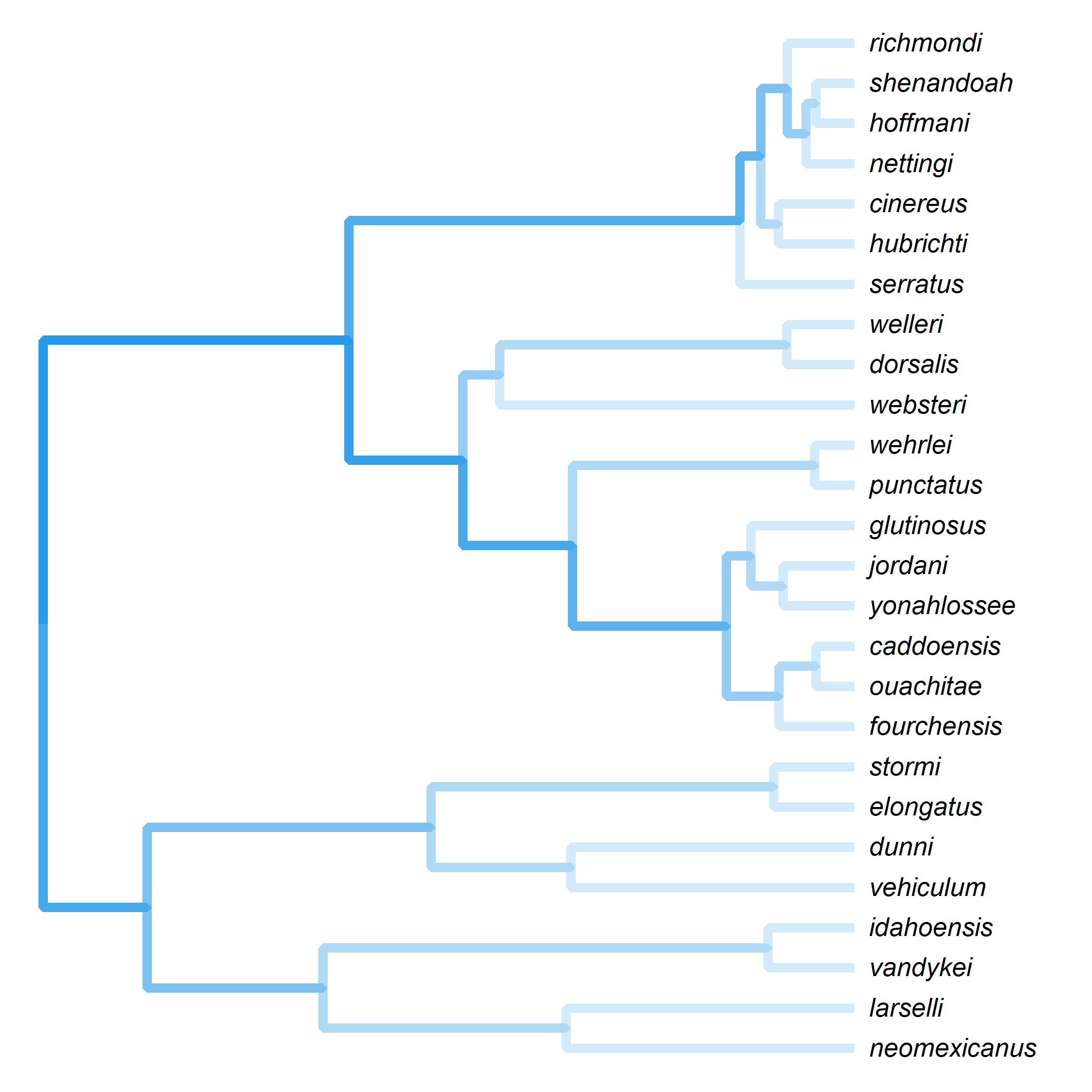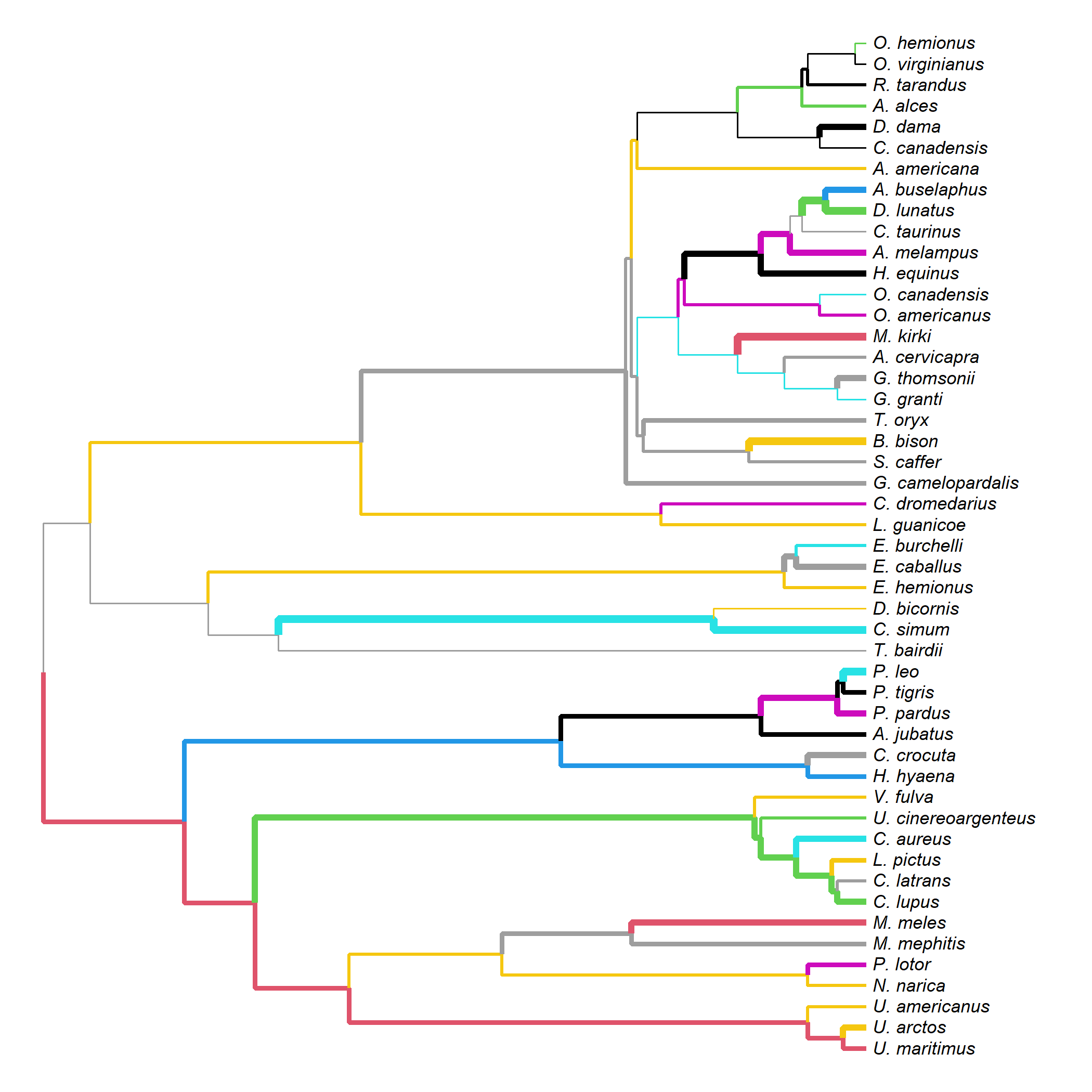## Tuesday, January 3, 2023

### Alternative algorithms for plotting phylogenies in R

Yesterday I posted an algorithm for plotting trees in which we drew every path from the root to any tip as a segmented line through each ancestral node.

This may not be the most computationally efficient way to plot a tree, but one possible advantage of this approach, I thought might be to avoid the overplotting at “joints” that occurs when each horizontal & vertical edge in the graphed tree is plotted as a separate line or segment. This overplotting only tends to become visible when we use semi-transparent colors – or if we use so-called “butt” line endings (`lend=1`).

``````library(phytools)
library(phangorn)
data(salamanders)
par(mfrow=c(1,2))
plotTree(salamanders,color=make.transparent("black",0.5),lwd=6,
ftype="i",mar=c(0.1,0.1,2.1,0.1))
plotTree(salamanders,color=palette(),lwd=6,ftype="i",
lend=1,mar=c(0.1,0.1,2.1,0.1))
``````Here’s a simple function based on my previous blog post.

``````lineTree<-function(...){
args<-list(...)
col<-if(!is.null(args\$color)) args\$color else par()\$fg
args\$color<-"transparent"
do.call(plotTree,args)
lwd<-if(!is.null(args\$lwd)) args\$lwd else par()\$lwd
obj<-get("last_plot.phylo",envir=.PlotPhyloEnv)
phy<-list(edge=obj\$edge,Nnode=obj\$Nnode,
tip.label=1:obj\$Ntip)
attr(phy,"class")<-"phylo"
for(i in 1:Ntip(phy)){
aa<-c(i,Ancestors(phy,i))
lines(obj\$xx[aa],obj\$yy[aa],col=col,type="s",
lwd=lwd)
}
}
par(lend=1,ljoin=2)
lineTree(salamanders,lwd=6,color=palette(),ftype="i")
``````We can see straight away that this plots much more cleanly – and even allows us to use a ‘beveled’ line joint (`ljoin=2`).

Unfortunately, this doesn’t obviate our overplotting problem as we’re actually overplotting all the edges of our graphs, except for the tips.

To see that, let’s set our color to be semi-transparent as follows.

``````par(lend=1,ljoin=2)
lineTree(salamanders,lwd=6,
color=make.transparent(color=palette(),0.2),
ftype="i")
``````As an alternative solution to the same problem, let’s just record the line segments that we’ve plotted, and then avoid overplotting them – graphing from an internal node to each tip.

To get an idea of how this might work, let’s plot each separately graphed line using a different color and line width. We should see that no/minimal overplotting occurs.

``````set.seed(7)
``````
``````lineTree.2<-function(...){
args<-list(...)
args\$color<-"transparent"
do.call(plotTree,args)
obj<-get("last_plot.phylo",envir=.PlotPhyloEnv)
nm<-nrow(obj\$edge)
draw<-1:max(obj\$edge)
phy<-list(edge=obj\$edge,Nnode=obj\$Nnode,
tip.label=1:obj\$Ntip)
attr(phy,"class")<-"phylo"
for(i in 1:Ntip(phy)){
aa<-c(i,intersect(Ancestors(phy,i),draw))
capture.output(aa<-c(aa,getParent(phy,
aa[length(aa)])))
xx<-obj\$xx[aa]
yy<-obj\$yy[aa]
lines(xx,yy,lwd=sample(1:5,1),
col=sample(palette(),1),
type="s")
draw<-setdiff(draw,aa)
Sys.sleep(0.1)
}
}
data(mammal.tree)
par(lend=1,ljoin=2)
lineTree.2(mammal.tree,fsize=0.7,ftype="i")
``````This code chunk also includes a `Sys.sleep` lag, causing lines to appear one-by-one instead of all at once, which (if animated) would look something like this.